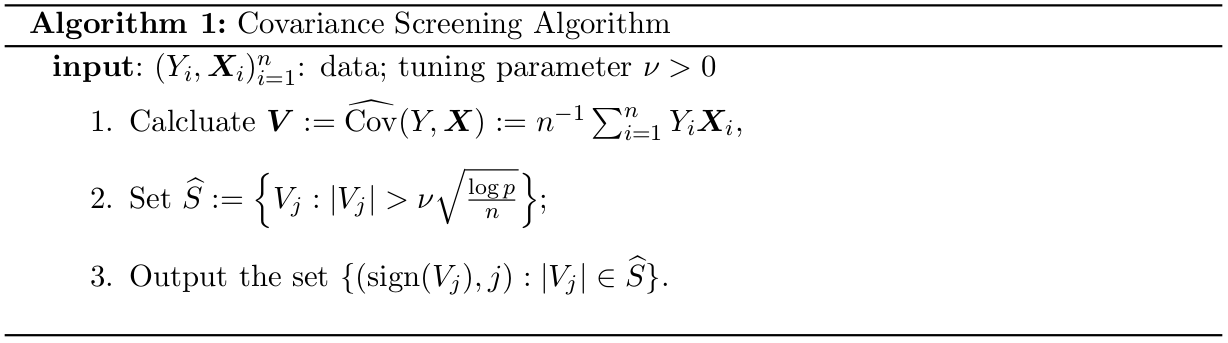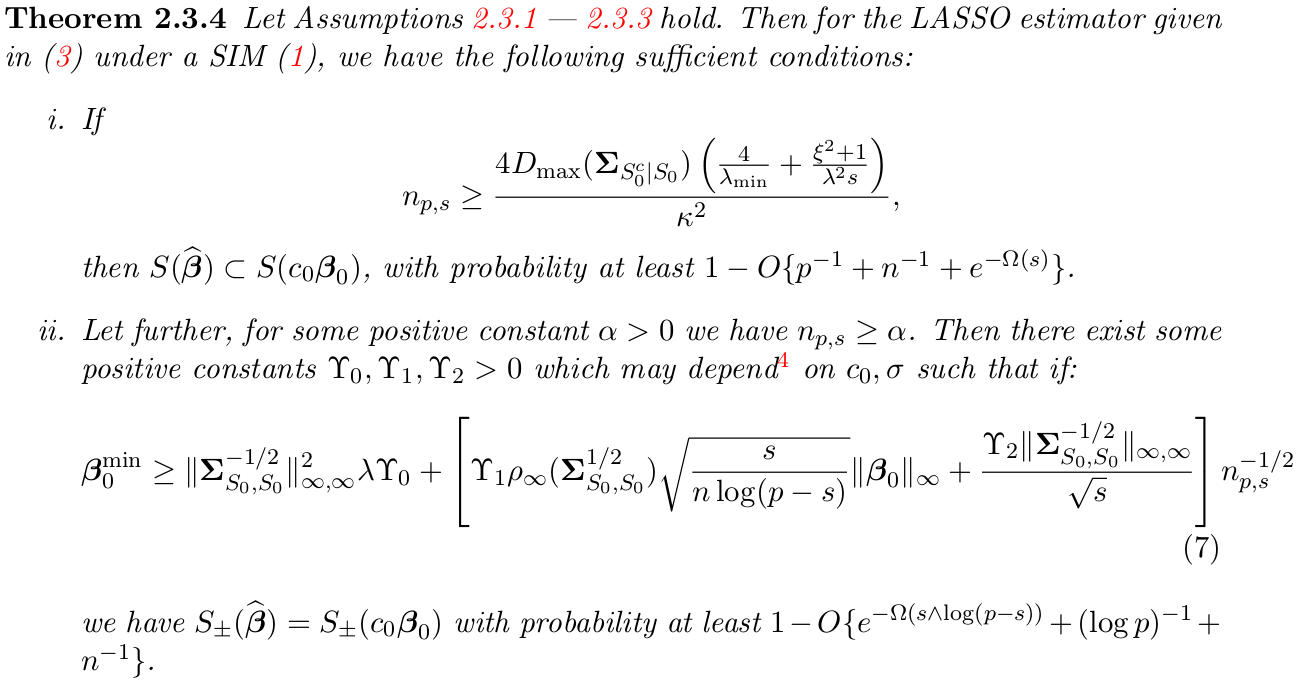# Least Squares for SIMs

##### Posted on Apr 15, 2019

In the last lecture of STAT 5030, Prof. Lin shared one of the results in the paper, Neykov, M., Liu, J. S., & Cai, T. (2016). L1-Regularized Least Squares for Support Recovery of High Dimensional Single Index Models with Gaussian Designs. Journal of Machine Learning Research, 17(87), 1–37., or say the start point for the paper—the following Lemma. Because it seems that the condition and the conclusion is completely same with Sliced Inverse Regression, except for a direct interpretation—the least square regression.

## Abstract

• Background: A certain class of certain single index models (SIMs) $Y=f(\bX_{p\times 1}^T\bbeta_0,\varepsilon)$, support recovery is impossible when $\bX\sim N(0,I_{p\times p})$ and a model complexity adjusted sample size is below a critical threshold.
• Current (2016) techniques: optimal algorithms based on Sliced Inverse Regression work provably under the assumption that the design $\bX$ comes from an i.i.d. Gaussian distribution.
• Proposal:
• analyze algorithms based on covariance screening and least squares with $L_1$ penalization
• demonstrate that they can also enjoy optimal (up to a scalar) rescaled sample size in terms of support recovery, albeit under slightly different assumptions on $f$ and $\varepsilon$ compared to the SIR based algorithms
• show more generally that LASSO succeeds in recovering the signed support of $\bbeta_0$ if $\bX\sim N(0,\bSigma)$, and the covariance $\bSigma$ satisfies the irrepresentable condition.
• the work extends existing results on the support recovery of LASSO for the linear model, to a more general class of SIMs.

## Introduction

The Lasso algorithm’s variable selection/support recovery capabilities under generalized linear models, have been extensively studied. However, much less is known under potential mis-specification of these commonly used models or under more general models.

Focus on recovering the support of the regression coefficients $\bbeta_0$ under a single index model (SIM):

$\begin{equation}\label{eq:sim} Y = f(\bX^T\bbeta_0,\varepsilon),\;\varepsilon \ind \bX\,, \end{equation}$

where

• $f$ and $\varepsilon$ are left unspecified
• $\bbeta_0^T\bSigma\bbeta_0=1$ for identifiability
• $E\bX=0$ and $\bSigma=E(\bX\bX^T)$
• $\bX\in\bbR^p\sim N(0,\bSigma)$
• $\bbeta_0$ is $s$-sparse with $s < p$

Aim to show that the standard least squares Lasso algorithm

$\hat\bbeta = \argmin_{\bbeta\in\bbR^p}\left\{\frac{1}{2n}\sum_{i=1}^n(Y_i-\bX_i^T\bbeta)^2+\lambda\Vert\bbeta\Vert_1\right\}$

can successfully recover the support of $\bbeta_0$ provided standard regularity conditions and that the model complexity adjusted effective sample size,

$n_{p,s} = n/\{s\log(p-s)\}$

is sufficiently large.

When $p$ is small, inference under a SIM has been studied extensively in the literature among many others. When $\X\sim N(0,\bSigma)$, it can be showed that $\argmin_\beta\{\sum_{i=1}^n(Y_i-\bX_i^T\bbeta)^2\}$ consistently estimates $\bbeta_0$ up to a scalar. When $\bbeta_0$ is sparse, the sparse sliced inverse regression procedure can be used to effectively recover $\bbeta_0$ under model \eqref{eq:sim} although their procedure requires a consistent estimator of $\bSigma^{-1/2}$.

In the high dimensional setting with diverging $p$,

• sparse SIM with an estimation framework using the PAC-Bayesian approach
• support recovery can be achieved when $p = O(n^k)$ under a SIM via optimizations in the form of
$\hat\bbeta = \argmin_{\bbeta\in\bbR^p}\frac{1}{2n}\sum_{i=1}^n(F_n(Y_i)-1/2-\bX_i^T\bbeta)^2 + \sum_{j=1}^pJ_\lambda(\beta_j)\,,$

where $J_\lambda$ is a penalty function and $F_n(x) = \frac 1n\sum_{i=1}^n1(Y_i\le x)$.

• regularized procedures for specific choices of $f$ and $Y$.

With $p$ potentially growing with $n$ exponentially and under a general SIM,

• someone proposed a non-parametric least squares with an equality $L_1$ constraint to handle simultaneous estimation of $\bbeta$ as well as $f$, but the support recovery properties are not investigated
• someone suggested a penalized approach, where they use a loss function related to Kendall’s tau correlation coefficient, they also establish the $L_2$ consistency for the coefficient $\bbeta$ but do not consider support recovery.
• someone analyzed two algorithms based on SIR under the assumption that $\bX\sim N(0,I_{p\times p})$, and demonstrated that they can uncover the support optimally in terms of the rescaled sample size.

## Main Results

Assume that the SIM \eqref{eq:sim} holds, $\bSigma = \bbE(\bX\bX^T) > 0$, and $\bX$ satisfies the linearity in expectation condition in the direction $\bbeta_0$ such that $\bbE[(\X^T\bbeta_0)^2]>0$. Then we have $\bSigma^{-1}\bbE(Y\bX)=c_0\bbeta_0$, where $c_0:=\bbE(Y\bX^T\bbeta_0)$. Obviously, $\bbE(Y\bX)=c_0\bbeta_0$ when $\bSigma = I_{p\times p}$.

Motivated by the special case of Lasso,

$\beta_j =\sign\left(n^{-1}\sum_{i=1}^nY_iX_{ij}\right)\left(\left\vert n^{-1}\sum_{i=1}^nY_iX_{ij} \right\vert - \lambda\right)_+$

consider the following simple covariance screening procedure.It can be showed that the algorithm recovers the support with probability approaching 1 provided that the effective sample size $n_{p,s}$ is sufficiently large under the normality assumption $\bX\sim N(0,I_{p\times p})$.

Then consider general $\bSigma$, that is, $\bX\sim N(0,\bSigma)$. Apply primal dual witness (PDW), get the main result,## Discussion

demonstrate that under a high dimensional SIM, $L_1$-regularized least squares, including a simplified covariance screening procedure under orthogonal design, is robust in terms of model selection consistency, in that it correctly recovers the support of the true regression parameter $\bbeta_0$ under specific conditions.

the results extend known results on the support recovery performance of LASSO under linear models to a much broader class of SIMs.

So the main point actually is the awareness of the lemma, which can treat the SIR as directly least squares?

Published in categories Note